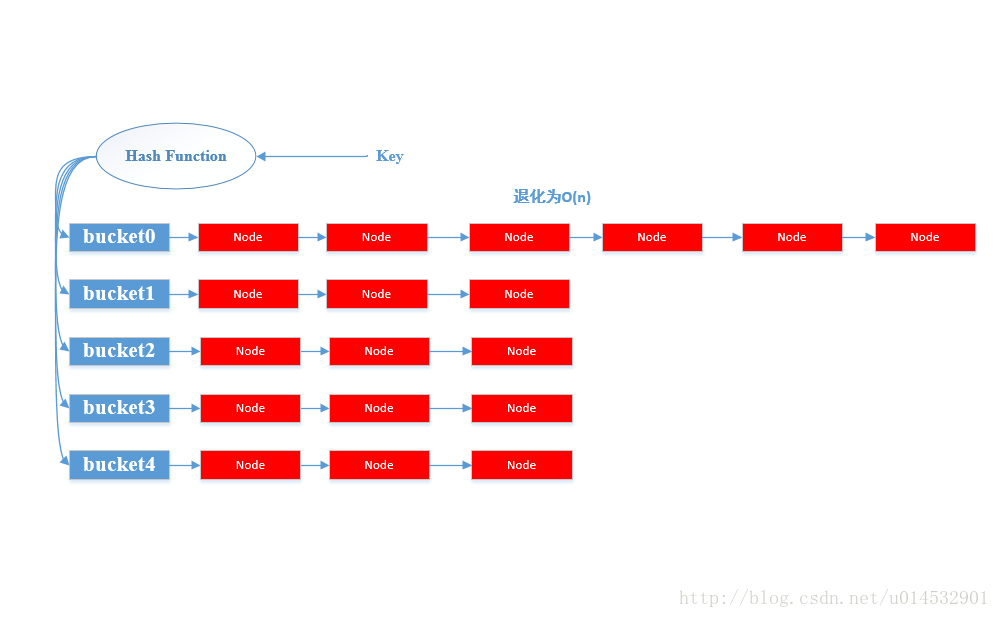# HashMap工作原理和扩容机制

2019/06/19 09:59

1. HashMap工作原理

HashMap作为优秀的Java集合框架中的一个重要的成员，在很多编程场景下为我们所用。HashMap作为数据结构散列表的一种实现，就其工作原理来讲单独列出一篇博客来讲都是不过分的。由于本文主要是简单总结其扩容机制，因此对于HashMap的实现原理仅做简单的概述。

HashMap内部实现是一个桶数组，每个桶中存放着一个单链表的头结点。其中每个结点存储的是一个键值对整体（Entry），HashMap采用拉链法解决哈希冲突（关于哈希冲突后面会介绍）。HashMap提供两个重要的基本操作，put(K, V)和get(K)。

当调用put操作时，HashMap计算键值K的哈希值，然后将其对应到HashMap的某一个桶(bucket)上；此时找到以这个桶为头结点的一个单链表，然后顺序遍历该单链表找到某个节点的Entry中的Key是等于给定的参数K；若找到，则将其的old V替换为参数指定的V；否则直接在链表尾部插入一个新的Entry节点。
对于get(K)操作类似于put操作，HashMap通过计算键的哈希值，先找到对应的桶，然后遍历桶存放的单链表通过比照Entry的键来找到对应的值。

2. HashMap扩容
2.1 HashMap的扩容时机

public HashMap(int initialCapacity, float loadFactor) ;

1

第一个参数：初始容量，指明初始的桶的个数；相当于桶数组的大小。
第二个参数：装载因子，是一个0-1之间的系数，根据它来确定需要扩容的阈值，默认值是0.75。

put(K, V)操作

public V put(K key, V value) {
if (key == null)
return putForNullKey(value);
int hash = hash(key);//计算键的hash值
int i = indexFor(hash, table.length);//通过hash值对应到桶位置
for (Entry<K,V> e = table[i]; e != null; e = e.next) {//顺序遍历桶外挂的单链表
Object k;
if (e.hash == hash && ((k = e.key) == key || key.equals(k))) {//注意这里的键的比较方式== 或者 equals()
V oldValue = e.value;
e.value = value;
e.recordAccess(this);
return oldValue;
}
}

modCount++;
return null;
}

/**
* Adds a new entry with the specified key, value and hash code to
* the specified bucket.  It is the responsibility of this
* method to resize the table if appropriate.
*
* Subclass overrides this to alter the behavior of put method.
*/
void addEntry(int hash, K key, V value, int bucketIndex) {
if ((size >= threshold) && (null != table[bucketIndex])) {//当size大于等于某一个阈值thresholdde时候且该桶并不是一个空桶；
/*这个这样说明比较好理解：因为size 已经大于等于阈值了，说明Entry数量较多，哈希冲突严重，那么若该Entry对应的桶不是一个空桶，这个Entry的加入必然会把原来的链表拉得更长，因此需要扩容；若对应的桶是一个空桶，那么此时没有必要扩容。*/
resize(2 * table.length);//将容量扩容为原来的2倍
hash = (null != key) ? hash(key) : 0;
bucketIndex = indexFor(hash, table.length);//扩容后的，该hash值对应的新的桶位置
}

createEntry(hash, key, value, bucketIndex);//在指定的桶位置上，创建一个新的Entry
}

/**
* Like addEntry except that this version is used when creating entries
* as part of Map construction or "pseudo-construction" (cloning,
* deserialization).  This version needn't worry about resizing the table.
*
* Subclass overrides this to alter the behavior of HashMap(Map),
*/
void createEntry(int hash, K key, V value, int bucketIndex) {
Entry<K,V> e = table[bucketIndex];
table[bucketIndex] = new Entry<>(hash, key, value, e);//链表的头插法插入新建的Entry
size++;//更新size
}

size
threshold

/**
* The number of key-value mappings contained in this map.
*/
transient int size;

/**
* The next size value at which to resize (capacity * load factor).
* @serial
*/
int threshold;

/**
* The load factor for the hash table.
*
* @serial
*/

size记录的是map中包含的Entry的数量

而threshold记录的是需要resize的阈值 且 threshold = loadFactor * capacity

capacity 其实就是桶的长度

threshold = (int)Math.min(newCapacity * loadFactor, MAXIMUM_CAPACITY + 1);
1

2.2 HashMap的扩容过程

/**
* Rehashes the contents of this map into a new array with a
* larger capacity.  This method is called automatically when the
* number of keys in this map reaches its threshold.
*
* If current capacity is MAXIMUM_CAPACITY, this method does not
* resize the map, but sets threshold to Integer.MAX_VALUE.
* This has the effect of preventing future calls.
*
* @param newCapacity the new capacity, MUST be a power of two;
*        must be greater than current capacity unless current
*        capacity is MAXIMUM_CAPACITY (in which case value
*        is irrelevant).
*/
void resize(int newCapacity) {
Entry[] oldTable = table;
int oldCapacity = oldTable.length;
if (oldCapacity == MAXIMUM_CAPACITY) {//最大容量为 1 << 30
threshold = Integer.MAX_VALUE;
return;
}

Entry[] newTable = new Entry[newCapacity];//新建一个新表
boolean oldAltHashing = useAltHashing;
useAltHashing |= sun.misc.VM.isBooted() &&
(newCapacity >= Holder.ALTERNATIVE_HASHING_THRESHOLD);
boolean rehash = oldAltHashing ^ useAltHashing;//是否再hash
transfer(newTable, rehash);//完成旧表到新表的转移
table = newTable;
threshold = (int)Math.min(newCapacity * loadFactor, MAXIMUM_CAPACITY + 1);
}

/**
* Transfers all entries from current table to newTable.
*/
void transfer(Entry[] newTable, boolean rehash) {
int newCapacity = newTable.length;
for (Entry<K,V> e : table) {//遍历同桶数组中的每一个桶
while(null != e) {//顺序遍历某个桶的外挂链表
Entry<K,V> next = e.next;//引用next
if (rehash) {
e.hash = null == e.key ? 0 : hash(e.key);
}
int i = indexFor(e.hash, newCapacity);//找到新表的桶位置;原桶数组中的某个桶上的同一链表中的Entry此刻可能被分散到不同的桶中去了，有效的缓解了哈希冲突。
e.next = newTable[i];//头插法插入新表中
newTable[i] = e;
e = next;
}
}
}

3. 补充
3.1 容量必须是2的幂

/**
* The default initial capacity - MUST be a power of two.
*/
static final int DEFAULT_INITIAL_CAPACITY = 16;

public HashMap(int initialCapacity, float loadFactor) {
if (initialCapacity < 0)
throw new IllegalArgumentException("Illegal initial capacity: " +
initialCapacity);
if (initialCapacity > MAXIMUM_CAPACITY)
initialCapacity = MAXIMUM_CAPACITY;
throw new IllegalArgumentException("Illegal load factor: " +

// Find a power of 2 >= initialCapacity 找到一个大于等于初始容量的且是2的幂的数作为实际容量
int capacity = 1;
while (capacity < initialCapacity)
capacity <<= 1;

threshold = (int)Math.min(capacity * loadFactor, MAXIMUM_CAPACITY + 1);
table = new Entry[capacity];
useAltHashing = sun.misc.VM.isBooted() &&
(capacity >= Holder.ALTERNATIVE_HASHING_THRESHOLD);
init();
}

/**
* Returns index for hash code h.
*/
static int indexFor(int h, int length) {
return h & (length-1);
}

https://bugs.java.com/bugdatabase/view_bug.do?bug_id=4631373中提出Java的%、/操作比&慢10倍左右，因此采用&运算会提高性能。

length为16，对应的二进制位(1 0000)

%操作：311 = 16*19 + 7；所以结果为7，二进制位(0111)；

&操作：(1 0011 0111) & (0111) = 0111 = 7, 二进制位(0111)

1 0011 0111 = (1 0011 0000) + (0111) = (1*2^4 + 1* 2^5 + 0*2^6 + 0*2^7 + 1*2^8 ) + 7 = 2^4*(1 + 2 + 0 + 0 + 16) + 7 = 16 * 19 + 7; 和%操作一致。

3.2 rehash

key1的hashcode为11111 10101，另一个key2的hashcode为00000 10101，很明显这两个hashcode不是一样的，甚至连相似性（例如海明距离）也是很远的。但是直接进行&操作得出的桶位置是同一个桶，这直接就产生了哈希冲突。

/**
* Retrieve object hash code and applies a supplemental hash function to the
* result hash, which defends against poor quality hash functions.  This is
* critical because HashMap uses power-of-two length hash tables, that
* otherwise encounter collisions for hashCodes that do not differ
* in lower bits. Note: Null keys always map to hash 0, thus index 0.
*/
final int hash(Object k) {
int h = 0;
if (useAltHashing) {
if (k instanceof String) {
return sun.misc.Hashing.stringHash32((String) k);
}
h = hashSeed;
}

h ^= k.hashCode();

// This function ensures that hashCodes that differ only by
// constant multiples at each bit position have a bounded
// number of collisions (approximately 8 at default load factor).
h ^= (h >>> 20) ^ (h >>> 12);
return h ^ (h >>> 7) ^ (h >>> 4);
}

1
3 收藏

### 作者的其它热门文章0 评论
3 收藏
1